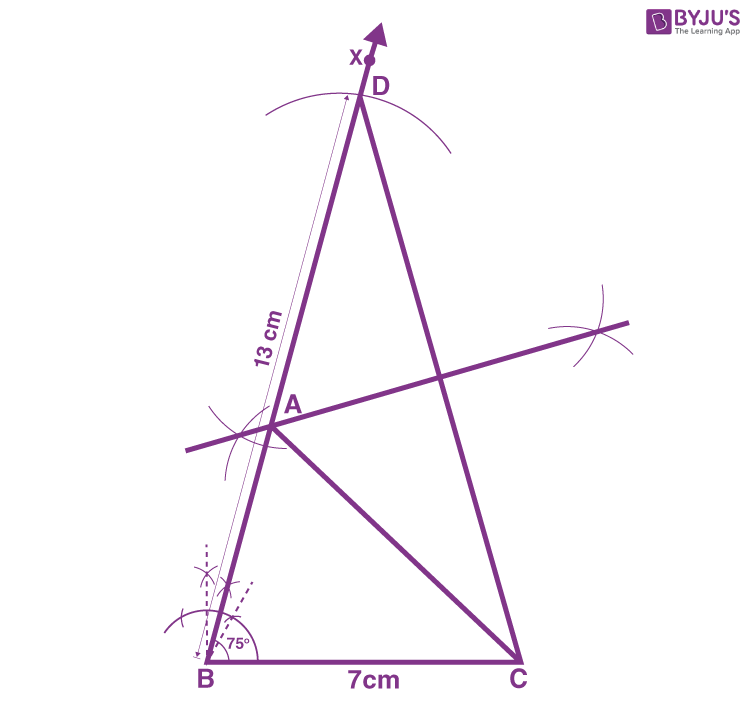# Construct a triangle ABC in which BC = 7cm, ∠B = 75° and AB + AC = 13 cm.Construction Procedure for the given parameters

1. Draw a line segment of base BC = 7 cm

2. Measure and draw ∠B = 75° and extend the ray BX

3. ?Using a compass and measure AB+AC = 13 cm.

4. Considerinh B as the centre, draw an arc at the point be D

5. Join DC

6. Now draw the perpendicular bisector of the line DC and the intersection point is taken as A.

7. Join AC

8. Therefore, ABC is the required triangle.